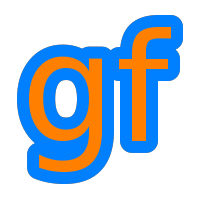Gamedev Framework (gf)  0.1.0 A C++11 framework for 2D games
gf::Range< T > Struct Template Reference

A half-open range of values. More...

#include <gf/Range.h>

## Classes

struct  Iterator
A range iterator. More...

## Public Member Functions

constexpr bool contains (T value) const noexcept
Check if a value is in a range. More...

constexpr Iterator begin () const noexcept
Get a begin iterator. More...

constexpr Iterator end () const noexcept
Get a end iterator. More...

constexpr T length () const noexcept
Get the length of the range. More...

constexpr bool isEmpty () const noexcept
Check if the range is empty. More...

constexpr bool isValid () const noexcept
Check is the range is valid. More...

## Public Attributes

T lo
The lower endpoint (included) More...

T hi
The higher endpoint (excluded) More...

## Detailed Description

### template<typename T> struct gf::Range< T >

A half-open range of values.

gf::Range represents a half-open range of values.

## Member Function Documentation

template<typename T >
 constexpr Iterator gf::Range< T >::begin ( ) const
inlinenoexcept

Get a begin iterator.

Returns
A begin iterator
end()
template<typename T >
 constexpr bool gf::Range< T >::contains ( T value ) const
inlinenoexcept

Check if a value is in a range.

Parameters
 value The value to test
Returns
True if the value is in the range
template<typename T >
 constexpr Iterator gf::Range< T >::end ( ) const
inlinenoexcept

Get a end iterator.

Returns
A end iterator
begin()
template<typename T >
 constexpr bool gf::Range< T >::isEmpty ( ) const
inlinenoexcept

Check if the range is empty.

Returns
True if the range is empty
isValid()
template<typename T >
 constexpr bool gf::Range< T >::isValid ( ) const
inlinenoexcept

Check is the range is valid.

Returns
True if the range is valid
isEmpty()
template<typename T >
 constexpr T gf::Range< T >::length ( ) const
inlinenoexcept

Get the length of the range.

Returns
The length of the range

## Member Data Documentation

template<typename T >
 T gf::Range< T >::hi

The higher endpoint (excluded)

template<typename T >
 T gf::Range< T >::lo

The lower endpoint (included)